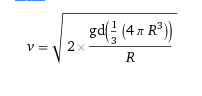# Question About the Escape Velocity from Different Mass Planets

lify34
Hi!

I have a question about escape velocity. If a planet is bigger and have a greater escape velocity than another planet. Do this effect the density of the bigger planet in any way? Or do we have to know the mass of the bigger planet to know if the density is larger or lower for this planet?

Homework Helper
Do you know an equation for the escape velocity? How are the terms in that equation related to the density?

lify34
Do you know an equation for the escape velocity? How are the terms in that equation related to the density?
V= sqrt ((2*G*M)/(R))

And M is volume * density. Then the density have to be lager, for the bigger planet with a greater escape velocity?

Homework Helper
How does M vary with R? Put this into the equation, and see how V varies with R for constant density.

lify34
How does M vary with R? Put this into the equation, and see how V varies with R for constant density.

I do not really understand ,as we do not know if they have the same density

Homework Helper
Assume they do, and see what you get. Compare your result with the hypothesis that the bigger planet must have greater density.

Staff Emeritus
Gold Member
I do not really understand ,as we do not know if they have the same density
Mass equals density x volume.
if we assume a spherical volume, volume = 4 pi R3/3
Thus M= 4 pi d R3/3
where d is the density.
Sub this for M in the escape velocity equation and see what you get.

lify34
I can see when I have the same density and only change radius R to a larger value, I get a larger value for escape velocity. Maybe it is hard to draw a conclusions from this as we do not know if the density is the same...

Staff Emeritus
Gold Member
I can see when I have the same density and only change radius R to a larger value, I get a larger value for escape velocity. Maybe it is hard to draw a conclusions from this as we do not know if the density is the same...
You can look up the radii, masses and densities of the planets in the Solar system, and you can calculate their escape velocities.

Gold Member
I think what he is asking is the other way around.
I think he's really asking if a bigger/more massive planet tends to be denser.
I think the escape velocity aspect is an inadvertent red herring.

lify34
I think what he is asking is the other way around.
I think he's really asking if a bigger/more massive planet tends to be denser.
I think the escape velocity aspect is an inadvertent red herring.
I mean in general. If a planet have a slightly faster escape velocity and is bigger than another planet. What can we say about the density of this bigger planet with a faster escape velocity? Is the density for this planet larger or lower compared to the planet that is smaller and have a less escape velocity?

2022 Award
What can we say about the density of this bigger planet with a faster escape velocity?
Not a lot if you don't suggest numbers. It could be more or less dense, or the same density.

Have you done the maths that has been suggested here? If so, post your formula relating density, size and escape velocity. Note that you can use LaTeX for maths - e.g. $$F=\frac{GMm}{r^2}$$See https://www.physicsforums.com/help/latexhelp/

•PeroK
lify34
That's what I thought, I need more information to be able to draw a conclusion.V is the escape velocity, g universal gravitational constant, d density and R the radius.

I

#### Attachments

Homework Helper
Gold Member
2022 Award
That's what I thought, I need more information to be able to draw a conclusion.
View attachment 238512
V is the escape velocity, g universal gravitational constant, d density and R the radius.

I

If person A is bigger and heavier than person B, then what can we say about their relative densities? And, as @Ibix points out, not a lot unless you put some numbers in.

Escape velocity depends on both density and size.

If, however, you have a smaller planet (A) with a greater escape velocity than a bigger planet (B), then you know that A is denser than B.

slappmunkey
Lify, remember that size, mass, and density are 3 different things.
If you have a 1'x'1' cube of styrofoam vs a 1'x1' cube of lead, the size is the same, but the mass and density are much more for the lead. Understanding that will answer your question indefinitely

Last edited by a moderator:
Staff Emeritus
Gold Member
Concrete examples :
Earth:
mass: 5.97e24 kg, density: 5.52 g/c3, escape velocity: 11.19 km/sec

Jupiter:
mass 1.9e27 kg, density: 1.33 g/c3, escape velocity: 59.5 km/sec

Staff Emeritus
Homework Helper
Gold Member
V= sqrt ((2*G*M)/(R))

And M is volume * density. Then the density have to be lager, for the bigger planet with a greater escape velocity?
No. If the average densities are the same, the escape velocity is directly proportional to the radius.

The volume of a sphere is given by ##\ \text{Volume} = \dfrac 4 3 \pi R^3 \,, \; ## so the mass, M, of a spherical planet of average density, ρ, is:
## M = \dfrac 4 3 \pi R^3 \,\rho ##​
Plug this into the expression for escape velocity, ##v,## to get ##\ v=\sqrt{2G \dfrac {4 \pi R^3}{3R} \,\rho}~, \; ## which simplifies to

##\ v=\sqrt{2G \dfrac {4 \pi }{3} \,} \; \sqrt \rho ~R \ \ . ##​
.
Look at the ratio of escape velocities for planets 1 and 2.

## \dfrac {v_2}{v_1} = \sqrt {\dfrac {\rho_2}{\rho_1} } \cdot \dfrac {R_2}{R_1} ##
.

•LURCH